# Plotting with TikZ, Part III: Plotting a function defined by a formula, and plotting functions that go through certain points

In the last post, I described how I use TikZ to create a graph that might otherwise be created freehand: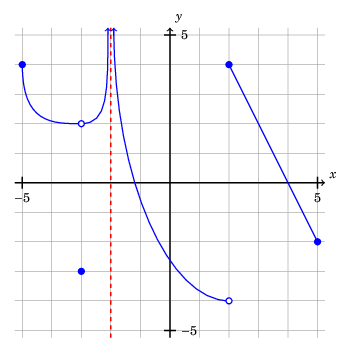In this post, I will describe one method for using TikZ to to plot a function defined by a formula, such as $$y = \left(2x^2 - x - 1\right)e^{-x}.$$ Then I will show two ways to make a function go through a certain point.

# Plotting from a formula

## Simple example

There are a number of ways to achieve this, and PGF actually includes the functionality to perform calculations in TeX. (For example, I have used the PGF pseudorandom number generator in graphics. Someone even made TikZ code to generate a random city skyline.) However, for efficiency sake, I prefer to have the calculations done outside TeX, in a more efficient computer language.

The trick is to use the gnuplot table import format. You simply have to generate a text file with a list of coordinates in the following form:

-1.250000 11.779907
-1.240000 11.456050
-1.230000 11.138839


This file contains the coordinates $(-1.25, 11.779907)$, $(-1.24, 11.456050)$, $(-1.23, 11.138839)$.

There are a number of ways to generate such a file. Since I like Python, I used the following (simple) Python script:

  1 2 3 4 5 6 7 8 9 10 11  #! /usr/bin/env python3 import numpy as np f = lambda x: (2*x**2 - x - 1)*np.exp(-x) xvals = np.arange(-1.25, 10.25, 0.01) with open("plot1.table", "wt") as outfile: for x in xvals: outfile.write("{:f} {:f}\n".format(x, f(x)))

Now, this curve can be imported into your TikZ illustration using \draw […] plot; in this case:

\draw[thick, blue, <->] plot[smooth] file {plot1.table};

Here is a complete example (with coordinate axes and everything):

  1 2 3 4 5 6 7 8 9 10 11 12 13 14 15 16 17 18 19 20 21 22 23 24 25 26 27 28 29 30 31 32 33 34  \documentclass[border=0.25cm]{standalone} \usepackage{tikz} \usepackage{fouriernc} \begin{document} \begin{tikzpicture}[yscale=0.5] \draw[gray] (-1.25, -1.25) grid (10.25, 12.25); % x-axis \draw[thick, black, ->] (-1.25, 0) -- (10.25, 0) node[anchor=south west] {$x$}; % x-axis tick marks \foreach \x in {-1, 10} \draw[thick] (\x, 0.2) -- (\x, -0.2) node[anchor=north] {$$\x$$}; % y-axis \draw[thick, black, ->] (0, -1.25) -- (0, 12.25) node[anchor=south west] {$y$}; % y-axis tick marks \foreach \y in {-1, 12} \draw[thick] (-0.1, \y) -- (0.1, \y) node[anchor=west] {$$\y$$}; % graph of function \draw[thick, blue, <->] plot[smooth] file {plot1.table}; \end{tikzpicture} \end{document}

And here is the output: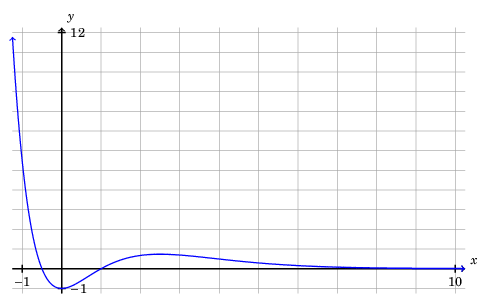In my Python script above, I carefully chose the set of inputs $x$ (np.arange(-1.25, 10.25, 0.01), i.e., every real number of the form $-1.25 + 0.01k, \; k \in \mathbb{Z}$ in the interval $[-1.25, 10.25)$) to keep $f(x) < 12$. This can also be handled computationally.

## Example involving asymptotes

For example, suppose I want to plot $y = r(x)$, where $$r(x) = \frac{6x^3 + 21x^2 - 21x - 36}{3x^3 + 3x^2 - 12x - 12},$$ with $-15.5 \leq x \leq 15.5$. Let’s additionally suppose I want to only see $y$-values with $-15.5 \leq y \leq 15.5$. (In other words, the “viewing rectangle” will be $[-15.5, 15.5] \times [-15.5, 15.5]$.)

Factoring the numerator and denominator, we have:

$$r(x) = \frac{6x^3 + 21x^2 - 21x - 36}{3x^3 + 3x^2 - 12x - 12}$$ $$\phantom{r(x)}= \frac{\left(x + 1\right) \left(2 x - 3\right) \left(3 x + 12\right)}{\left(x + 1\right) \left(x + 2\right) \left(3 x - 6\right)}$$ $$\phantom{r(x)}= \frac{\left(2 x - 3\right) \left(3 x + 12\right)}{\left(x + 2\right) \left(3 x - 6\right)}, \; x \not = -1.$$

As you can see, we will have vertical asymptotes at $x = -2$ and $x = 2$, and a hole at $x = -1$. There will also be a horizontal asymptote at $y = 2$.

To handle the asymptotes, we plot the function $$\hat r(x) = \frac{\left(2 x - 3\right) \left(3 x + 12\right)}{\left(x + 2\right) \left(3 x - 6\right)}$$ along three intervals:

• $[-15.5, -2)$,
• $(-2, 2)$, and
• $(2, 15.5]$

(Actually, for each of the above intervals $I$, we plot something approximating $\left\{(x, \hat r(x)): x \in I \text{ and } |\hat r(x)|\leq 16\right\}$.)

Here is the Python script:

  1 2 3 4 5 6 7 8 9 10 11 12 13 14 15 16 17  #! /usr/bin/env python3 import numpy as np r = lambda x: (2*x - 3)*(3*x + 12)/((x + 2)*(3*x - 6)) xvals = [ np.arange(-15.5, -2, 0.01), np.arange(-2.01, 2, 0.01), np.arange(2.01, 15.6, 0.01) ] for idx, interval in enumerate(xvals): with open("plot2_{}.table".format(idx), "wt") as outfile: for x in interval: if abs(r(x)) <= 16: outfile.write("{:f} {:f}\n".format(x, r(x)))

Here is the corresponding LaTeX code:

  1 2 3 4 5 6 7 8 9 10 11 12 13 14 15 16 17 18 19 20 21 22 23 24 25 26 27 28 29 30 31 32 33 34 35 36 37 38 39 40 41 42 43 44 45 46  \documentclass[border=0.25cm]{standalone} \usepackage{tikz} \usepackage{fouriernc} \begin{document} \begin{tikzpicture}[scale=0.5] % Scale keeps the graphic small \draw[gray] (-15.5, -15.5) grid (15.5, 15.5); % x-axis \draw[thick, black, ->] (-15.5, 0) -- (15.5, 0) node[anchor=south west] {$x$}; % x-axis tick marks \foreach \x in {-15, -10, -5, 5, 10, 15} \draw[thick] (\x, 0.5) -- (\x, -0.5) node[anchor=north] {$$\x$$}; % y-axis \draw[thick, black, ->] (0, -15.5) -- (0, 15.5) node[anchor=south west] {$y$}; % y-axis tick marks \foreach \y in {-15, -10, -5, 5, 10, 15} \draw[thick] (-0.5, \y) -- (0.5, \y) node[anchor=west] {$$\y$$}; % graph of function \draw[thick, blue, <->] plot[smooth] file {plot2_0.table}; \draw[thick, blue, <->] plot[smooth] file {plot2_1.table}; \draw[thick, blue, <->] plot[smooth] file {plot2_2.table}; % hole at (-1, 5) \draw[thick, blue, fill=white] (-1, 5) circle (2.5mm); % vertical asymptotes \draw[thick, dashed, red] (-2, -15.5) -- (-2, 15.5); \draw[thick, dashed, red] (2, -15.5) -- (2, 15.5); % horizontal asymptote \draw[thick, dashed, red] (-15.5, 2) -- (15.5, 2); \end{tikzpicture} \end{document}

And here is the result: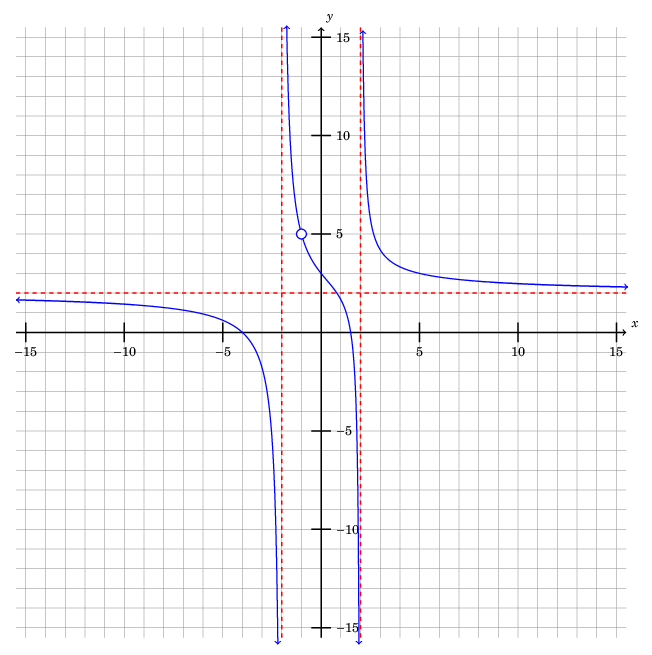# Plotting functions that go through certain points: two ways

Suppose I want to plot a function that go through certain points, say:

• $(-4, 2)$,
• $(-1, 6)$,
• $(2, 3)$, and
• $(4, 5)$.

I want the function to be differentiable.

## Method one: Bézier curves

Recall from the last post that a (cubic) Bézier curve is specified by four points:

• A start point ($(x_0, y_0)$ in the diagram below)
• Two control points ($(x_1, y_1)$ and $(x_2, y_2)$ in the diagram below)
• An end point ($(x_3, y_3)$ in the diagram below)

The syntax is: \draw ($x_0, y_0$) .. controls ($x_1, y_1$) and ($x_2, y_2$) .. ($x_3, y_3$);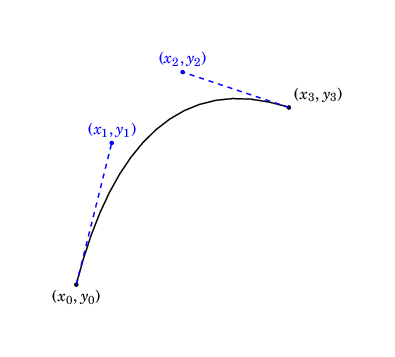Now, we can combine multiple Bézier curves into a single \draw. For example, \draw ($x_0, y_0$) .. controls ($x_1, y_1$) and ($x_2, y_2$) .. ($x_3, y_3$) .. controls ($x_4, y_4$) and ($x_5, y_5$) .. ($x_6, y_6$); will produce: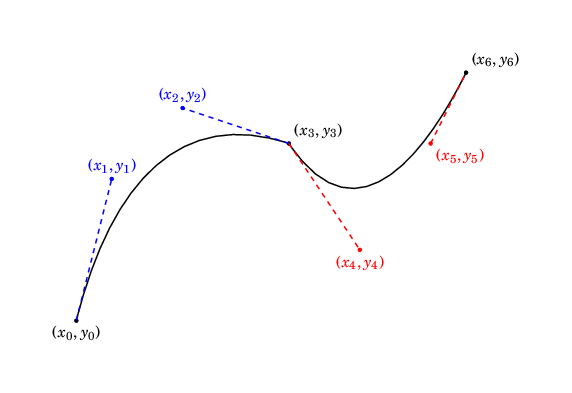In general, this curve will not necessarily be differentiable. We can get differentiability by ensuring that $(x_2, y_2)$, $(x_3, y_3)$ and $(x_4, y_4)$ are colinear (i.e., the end of the first Bézier curve has the same derivative as the beginning of the second) with $x_2 \not = x_3$: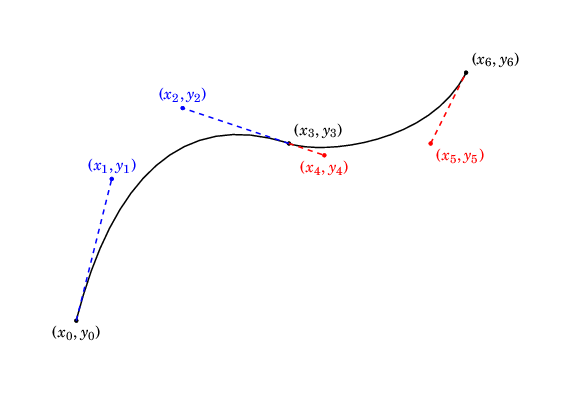Keeping these principles in mind, we can plot a function going through the desired points with:

  1 2 3 4 5 6 7 8 9 10 11 12 13 14 15 16 17 18 19 20 21 22 23 24 25 26 27 28 29 30 31 32 33 34 35 36 37 38 39  \documentclass[border=0.25cm]{standalone} \usepackage{tikz} \usepackage{fouriernc} \begin{document} \begin{tikzpicture}[scale=0.5] % Scale keeps the graphic small \draw[gray] (-5.25, 0) grid (5.25, 8.25); % x-axis \draw[thick, black, ->] (-5.25, 0) -- (5.25, 0) node[anchor=south west] {$x$}; % x-axis tick marks \foreach \x in {-5, 5} \draw[thick] (\x, 0.25) -- (\x, -0.25) node[anchor=north] {$$\x$$}; % y-axis \draw[thick, black, ->] (0, 0) -- (0, 8.25) node[anchor=south west] {$y$}; % y-axis tick marks \foreach \y in {4, 8} \draw[thick] (-0.25, \y) -- (0.25, \y) node[anchor=west] {$$\y$$}; % graph of function \draw[thick, blue, <->] (-5.25, -0.5) .. controls (-4, 0) and (-5, 0) .. (-4, 2) .. controls (-3.5, 3) and (-3, 6) .. (-1, 6) .. controls (1, 6) and (1.75, 4) .. (2, 3) .. controls (2.25, 2) and (3, 2) .. (4, 5) .. controls (4.5, 6.5) and (4.5, 7) .. (5.25, 8); \end{tikzpicture} \end{document}

Here is the output:## Method two: Polynomial interpolation

SciPy’s interpolation functionality can find a smooth function that passes through an arbitrary list of points. Here is an example of a Python script to produce such a function and create a table in the necessary format:

  1 2 3 4 5 6 7 8 9 10 11 12 13 14 15 16 17 18 19 20 21 22 23  #! /usr/bin/env python3 import numpy as np import scipy.interpolate coords = [(-10, 8), (-4, 2), (-1, 6), (2, 3), (4, 5), (10, 1)] # Since our goal is to plot from x = -5.25 to x = 5.25, # we need to specify some points beyond that interval on both sides. xcoords, ycoords = zip(*coords) # This produces a seperate list of x-coordinates and y-coordinates f = scipy.interpolate.interp1d(xcoords, ycoords, kind="cubic") xvals = np.arange(-5.25, 5.25, 0.01) yvals = f(xvals) outcoords = zip(xvals, yvals) # This produces a list of coordinate pairs with open("plot3.table", "wt") as outfile: for pair in outcoords: outfile.write("{:f} {:f}\n".format(pair, pair))

Note that if we had set coords = [(-4, 2), (-1, 6), (2, 3), (4, 5)], then the resulting function would not have had a defined value outside of the interval $[-4, 4]$.

To plot, we use the following tikzpicture:

  1 2 3 4 5 6 7 8 9 10 11 12 13 14 15 16 17 18 19 20 21 22 23 24 25 26 27 28 29 30 31 32 33 34  \documentclass[border=0.25cm]{standalone} \usepackage{tikz} \usepackage{fouriernc} \begin{document} \begin{tikzpicture}[scale=0.5] % Scale keeps the graphic small \draw[gray] (-5.25, 0) grid (5.25, 8.25); % x-axis \draw[thick, black, ->] (-5.25, 0) -- (5.25, 0) node[anchor=south west] {$x$}; % x-axis tick marks \foreach \x in {-5, 5} \draw[thick] (\x, 0.25) -- (\x, -0.25) node[anchor=north] {$$\x$$}; % y-axis \draw[thick, black, ->] (0, 0) -- (0, 8.25) node[anchor=south west] {$y$}; % y-axis tick marks \foreach \y in {4, 8} \draw[thick] (-0.25, \y) -- (0.25, \y) node[anchor=west] {$$\y$$}; % graph of function \draw[thick, blue, <->] plot[smooth] file {plot3.table}; \end{tikzpicture} \end{document}

Here is the result: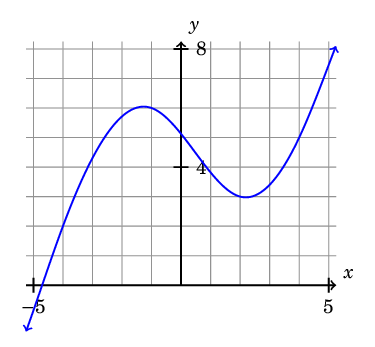As we have seen, creating plots with TikZ allows you a deep amount of control over the final result, and can be especially powerful when combined with a programming language such as Python.